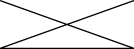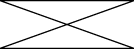# Drawing Arbitrary Shapes

You have already learned how to draw most of shapes represented in the `java.awt.geom` package. To create more complicated geometry, such as polygons, polylines, or stars you use another class from this package, `GeneralPath`.

This class implements the `Shape` interface and represents a geometric path constructed from lines, and quadratic and cubic curves. The three constructors in this class can create the `GeneralPath` object with the default winding rule (`WIND_NON_ZERO`), the given winding rule (`WIND_NON_ZERO` or `WIND_EVEN_ODD`), or the specified initial coordinate capacity. The winding rule specifies how the interior of a path is determined.

```public void paint (Graphics g) {
Graphics2D g2 = (Graphics2D) g;
...
}
```

To create an empty `GeneralPath` instance call `new GeneralPath()` and then add segments to the shape by using the following methods:

• `moveTo(float x, float y)` – Moves the current point of the path to the given point
• `lineTo(float x, float y)` – Adds a line segment to the current path
• `quadTo(float ctrlx, float ctrly, float x2, floaty2)` – Adds a quadratic curve segment to the current path
• `curveTo(float ctrlx1, float ctrly1, float ctrlx2, float ctrly2, float x3, floaty3)` – Adds a cubic curve segment to the current path
• `closePath()` – Closes the current path

The following example illustrates how to draw a polyline by using `GeneralPath`:

 ```// draw GeneralPath (polyline) int x2Points[] = {0, 100, 0, 100}; int y2Points[] = {0, 50, 50, 0}; GeneralPath polyline = new GeneralPath(GeneralPath.WIND_EVEN_ODD, x2Points.length); polyline.moveTo (x2Points, y2Points); for (int index = 1; index < x2Points.length; index++) { polyline.lineTo(x2Points[index], y2Points[index]); }; g2.draw(polyline); ```This example illustrates how to draw a polygon by using `GeneralPath`:

 ```// draw GeneralPath (polygon) int x1Points[] = {0, 100, 0, 100}; int y1Points[] = {0, 50, 50, 0}; GeneralPath polygon = new GeneralPath(GeneralPath.WIND_EVEN_ODD, x1Points.length); polygon.moveTo(x1Points, y1Points); for (int index = 1; index < x1Points.length; index++) { polygon.lineTo(x1Points[index], y1Points[index]); }; polygon.closePath(); g2.draw(polygon); ```Note that the only difference between two last code examples is the `closePath()` method. This method makes a polygon from a polyline by drawing a straight line back to the coordinates of the last `moveTo`.

To add a specific path to the end of your `GeneralPath` object you use one of the `append()` methods. The `ShapesDemo2D.java` code example contains additional implementations of arbitrary shapes.